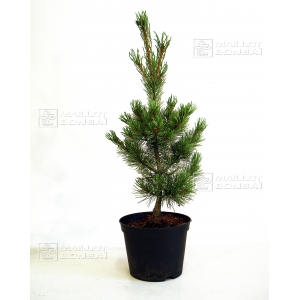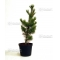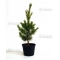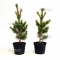##### The Japanese Bonsai specialist
Direct order Contact Help / Services Newsletter# Pinus pentaphylla 'blue lou'

Pinus pentaphylla "ryu ju"ref. : 1187395,00

Available quantity : 3Order

###### Description

Tree produced by grafting.

Size 60 cm.

Sold in a 20 litre pot.

A dwarf variety, pinus pentaphylla 'Blue Lou' is an ideal species for creating small to mid-sized bonsai. The needles are very small, appearing green in the spring and blue all summer, resulting in the most beautiful effect. We recommend cleaning it annually, getting rid of any dead needles on the branches.

Likes sun/mid-shade and fertilising with Biogold once a month until the end of October.

Potted in october 2022 don't touch the roots ball before spring 2023.

#blue 4.4 #pentaphylla 4.1 #pinus 3.5 #october 2.7 #needles 2.7 #spring 2.6 #trees 2.5 #small 2.5 #young 2.5 #fertilising 2.1

Formule
(( ROUND((CHAR_LENGTH(b.article_nom)-CHAR_LENGTH(REPLACE(b.article_nom, 'blue', '')))/LENGTH('blue')) + ROUND((CHAR_LENGTH(b.article_description)-CHAR_LENGTH(REPLACE(b.article_description, 'blue', '')))/LENGTH('blue')) ) * 4.4) + (( ROUND((CHAR_LENGTH(b.article_nom)-CHAR_LENGTH(REPLACE(b.article_nom, 'pentaphylla', '')))/LENGTH('pentaphylla')) + ROUND((CHAR_LENGTH(b.article_description)-CHAR_LENGTH(REPLACE(b.article_description, 'pentaphylla', '')))/LENGTH('pentaphylla')) ) * 4.1) + (( ROUND((CHAR_LENGTH(b.article_nom)-CHAR_LENGTH(REPLACE(b.article_nom, 'pinus', '')))/LENGTH('pinus')) + ROUND((CHAR_LENGTH(b.article_description)-CHAR_LENGTH(REPLACE(b.article_description, 'pinus', '')))/LENGTH('pinus')) ) * 3.5) + (( ROUND((CHAR_LENGTH(b.article_nom)-CHAR_LENGTH(REPLACE(b.article_nom, 'needles', '')))/LENGTH('needles')) + ROUND((CHAR_LENGTH(b.article_description)-CHAR_LENGTH(REPLACE(b.article_description, 'needles', '')))/LENGTH('needles')) ) * 2.7) + (( ROUND((CHAR_LENGTH(b.article_nom)-CHAR_LENGTH(REPLACE(b.article_nom, 'october', '')))/LENGTH('october')) + ROUND((CHAR_LENGTH(b.article_description)-CHAR_LENGTH(REPLACE(b.article_description, 'october', '')))/LENGTH('october')) ) * 2.7) + (( ROUND((CHAR_LENGTH(b.article_nom)-CHAR_LENGTH(REPLACE(b.article_nom, 'spring', '')))/LENGTH('spring')) + ROUND((CHAR_LENGTH(b.article_description)-CHAR_LENGTH(REPLACE(b.article_description, 'spring', '')))/LENGTH('spring')) ) * 2.6) + (( ROUND((CHAR_LENGTH(b.article_nom)-CHAR_LENGTH(REPLACE(b.article_nom, 'small', '')))/LENGTH('small')) + ROUND((CHAR_LENGTH(b.article_description)-CHAR_LENGTH(REPLACE(b.article_description, 'small', '')))/LENGTH('small')) ) * 2.5) + (( ROUND((CHAR_LENGTH(b.article_nom)-CHAR_LENGTH(REPLACE(b.article_nom, 'fertilising', '')))/LENGTH('fertilising')) + ROUND((CHAR_LENGTH(b.article_description)-CHAR_LENGTH(REPLACE(b.article_description, 'fertilising', '')))/LENGTH('fertilising')) ) * 2.1) + (( ROUND((CHAR_LENGTH(b.article_nom)-CHAR_LENGTH(REPLACE(b.article_nom, 'beautiful', '')))/LENGTH('beautiful')) + ROUND((CHAR_LENGTH(b.article_description)-CHAR_LENGTH(REPLACE(b.article_description, 'beautiful', '')))/LENGTH('beautiful')) ) * 1.9) + (( ROUND((CHAR_LENGTH(b.article_nom)-CHAR_LENGTH(REPLACE(b.article_nom, 'resulting', '')))/LENGTH('resulting')) + ROUND((CHAR_LENGTH(b.article_description)-CHAR_LENGTH(REPLACE(b.article_description, 'resulting', '')))/LENGTH('resulting')) ) * 1.9)

## Secure payment## Delivery

Our logistic partners :04 74 55 23 48
Pépinière MAILLOT-BONSAÏ
Le Bois Frazy
01990 RELEVANT - FRANCE
on appointment